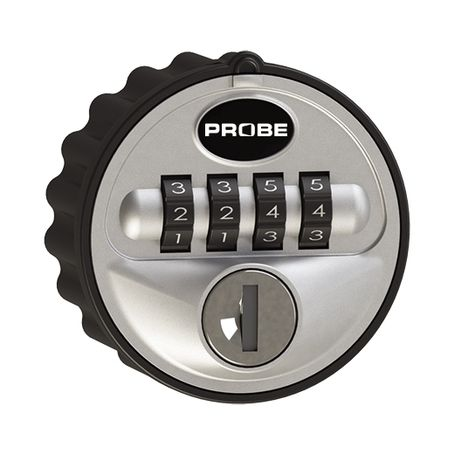# Practice on Toph

Participate in exhilarating programming contests, solve unique algorithm and data structure challenges and be a part of an awesome community.

# Combination Lock

By Sherlock221b · Limits 1s, 512 MB

Imagine a Combination Lock -If you set the numbers to a preset combination (passcode), the lock will open.

But our lock has a flaw. It has a tolerance value of K.

Imagine K = 2 and passcode is 1234. Then all the numbers from 1232 from 1236 will open the lock. Basically, a number x can open the lock if ABS(x-passcode) <= K (here ABS means Absolute Value).

In our picture, the lock has 4 digits. This means it can accomodate passcodes from 0000 to 9999. If a lock has 1 digit, it can accommodate passcodes from 0 to 9. If it has 2 digits, it can accommodate passcodes from 00 to 99 and so on.

A vault has N such locks attached to it. Lock i (1 <= i <= N) has di digits and its tolerance value is Ki. After you insert passcode in all of the locks, you will press a button attached to the vault. If the passcode inserted in all of the locks are correct, the vault will open.

Note that, a single lock will not open individually if you put correct passcode in it. The vault will only open after pressing the button when all locks have been inserted a correct passcode. So if you insert correct passcode in a lock, and incorrect passcode in some other lock, you have no way of knowing that you inserted correct passcode in some lock, because no change will be visible to the eye.

You don’t know the passcodes and want to open the vault. You can try opening the vault by setting the combinations of locks as many times as you want. What is the minimum number of times you need to press the button in order to open the vault in the worst case scenario? In other words, what is the minimum number of attempts after which the vault must open, irrespective of what the passcodes are? Since the answer can be huge, print the answer modulo 1000000007 (109+7). Please note that you need to minimize the number of times you need to press the button, not the mod value.

## Input

The first line of input contains an integer T which denotes the number of test cases.

The first line of each test case contains a single integer N denoting the number of locks. The following N lines describe the N locks. Each of these N lines contains two space separated integers di and Ki which denote the number of digits and the tolerance value of i-th lock respectively.

Constraints:

1 <= T <= 100

1 <= N <= 100

1 <= di <= 9

0 <= Ki <= 50

## Output

For each test case, print a single line in this format, “Case X: C” without the quotes, where X is the test case number and C is the minimum number of times you need to press the button attached to the vault in the worst case scenario modulo 1000000007 (109+7).

Follow the sample outputs for better understanding.

## Sample

InputOutput
8
1
1 0
1
2 0
1
1 1
1
2 2
1
1 50
2
1 0
1 0
2
2 2
2 2
5
9 0
8 1
9 1
9 0
7 4

Case 1: 10
Case 2: 100
Case 3: 4
Case 4: 20
Case 5: 1
Case 6: 100
Case 7: 400
Case 8: 849567876


Explanation:

For the first case, there is only one lock with a single digit and the tolerance value, K = 0 (which means you must insert the exact passcode to open the lock). Therefore, you need to try all 10 combinations (from 0 to 9) to ensure that the vault will open.

In the second case, there is only one lock with 2 digits and K = 0. So you need to try 100 different combinations (from 00 to 99) to ensure that the vault will open.

In the third case, there is only one lock with a single digit but this time, the tolerance value, K = 1. It can be shown that only 4 attempts are sufficient to ensure that the vault will open in this case.

In the fourth case, there is a single lock which has 2 digits and K = 2. So there are 100 different combinations possible on this lock (from 00 to 99). Only 20 of them are sufficient to ensure that the vault will open.

In the sixth case, there are two different locks. Both of them have only one digit and K = 0. So you need to try 100 different combinations in this case to ensure that the vault will open.

•### Statistics

95% Solution Ratio

notredLightest, 0 B

u1804014ayeshabanuShortest, 345B

### Submit